# KSEEB Solutions for Class 9 Maths Chapter 3 Lines and Angles Ex 3.3

In this chapter, we provide KSEEB SSLC Class 9 Maths Chapter 3 Lines and Angles Ex 3.3 for English medium students, Which will very helpful for every student in their exams. Students can download the latest KSEEB SSLC Class 9 Maths Chapter 3 Lines and Angles Ex 3.3 pdf, free KSEEB SSLC Class 9 Maths Chapter 3 Lines and Angles Ex 3.3 pdf download. Now you will get step by step solution to each question.

## Karnataka Board Class 9 Maths Chapter 3 Lines and Angles Ex 3.3

Question 1.
In Fig. 3.39, sides QP and RQ of ∆PQR are produced to points S and T respectively. If ∠SPR = 135° and ∠PQT = 110°, find ∠PRQ.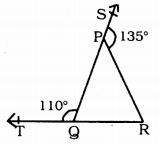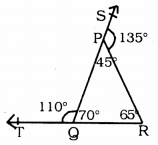Arms of the ∆PQR QP and RP are produced to S and T and ∠PQT = 110°, ∠SPR = 135°. ∠PRQ =?
Straight-line PR is on straight line SQ.
∠SPR and ∠RPQ are Adjacent angles.
∴ ∠SPR + ∠RPQ = 180°
135 + ∠RPQ = 180°
∴ ∠RPQ =180 – 135
∠RPQ = 45° (i)
Similarly QP straight line is on straight line TR.
∠TQP and ∠PQR are Adjacent angles.
∴ ∠RQP + ∠PQR = 180°
110 + ∠PQR = 180°
∠PQR = 180 – 110
∴ ∠PQR = 70°
Now, in ∆PQR,
∠QPR + ∠PQR + ∠PRQ = 180°
45 + 70 + ∠PRQ = 180°
115 + ∠PRQ = 180°
∠PRQ = 180 – 115
∴ ∠PRQ = 65°.

Question 2.
In Fig. 3.40, ∠X = 62°. ∠XYZ = 54°. If YO and ZO are the bisectors of ∠XYZ and ∠XZY respectively of ∆XYZ, find ∠OZY and ∠YOZ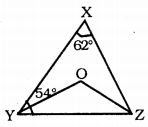In this figure, ∠X = 62°
∠XYZ = 54°
In ∆XYZ, YO and ZO are angular bisectors of ∠XYZ and ∠XZY.
Then, ∠OZY =?
∠OYZ =?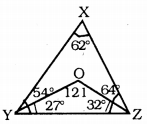In ∆XYZ
∠X + ∠Y + ∠Z = 180°
62 + 54 + ∠Z= 180
116 + ∠Z= 180
∠Z= 180- 116
∴ ∠Z = 64°
YO is the angular bisector of ∠Y
∴ ∠OYZ = 542 = 27°
ZO is the angular bisector of ∠Z
∴ ∠OZY = 642 = 32°
∴ ∠OZY = 32°
Now, in ∆OYZ,
∠OYZ + ∠OZY + ∠YOZ = 180°
27 + 32 + ∠YOZ = 180
59 + ∠YOZ = 180
∠YOZ = 180 – 59
∴∠YOZ = 121°
∴ ∠OZY = 32°
∠YOZ = 121°

Question 3.
In Fig. 3.41, if AB||DE, ∠BAC = 35° and ∠CDE = 53°, find ∠DCE.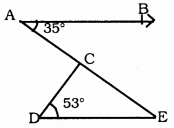If AB || DE, ∠BAC = 35, ∠CDE = 53 then ∠DCE = ?
AB || DE, AE is the bisector.
∴∠BAC = ∠DEC = 35° (∵ Alternate angles)
∴∠DEC= 35°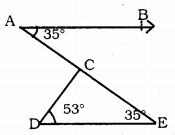Now in ∆CDE,
∠DCE + ∠CDE + ∠CED = 180°
∠DCE + 53 + 35 = 180
∠DCE + 88 = 180
∠DEC = 180 – 88
∴ ∠DCE = 92°.

Question 4.
In Fig. 3.42, if lines PQ and RS intersect at point T, such that ∠PRT = 40°, ∠RPT = 95° and ∠TSQ = 75°, find ∠SQT.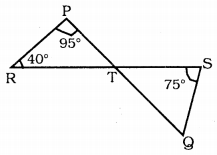PQ and RS straight lines intersect at T.
If ∠PRT = 40°, ∠RPT = 95°, and ∠TSQ = 75°, then ∠SQT =?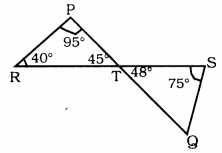In ∆PRT,
∠RPT + ∠PRT + ∠PTR = 180°
95 + 40 + ∠PTR = 180°
135 + ∠PTR = 180
∠PTR = 180 – 135
∴ ∠PTR = 45°
∠PTR = ∠STQ = 45° (∵ Vertically opposite angles)
In ∆TSQ,
∠STQ + ∠TSQ + ∠SQT =180
45 + 75 + ∠SQT = 180
120 + ∠SQT = 180
∴∠SQT = 180 – 120
∴ ∠SQT = 60°.

Question 5.
In Fig. 3.43, PQ ⊥ PS, PQ || SR, ∠SQR = 28° and ∠QRT = 65°. then find the value of x and y.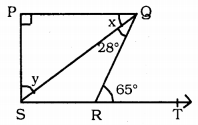PQ ⊥ PS, PQ ⊥ SR, ∠SQR = 28°, ∠QRT = 65°, Then x = ?, y = ?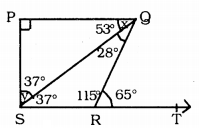Solution: ∠QRT + ∠QRS = 180° (∵ Linear pairs)
65 + ∠QRS = 180
∠QRS = 180 – 65
∴∠QRS =115°
In ∆SRQ,
∠QRS + ∠SQR + ∠RSQ = 180°
115 + 28 + ∠RSQ = 180
∴∠RSQ =180 – 143
∴∠RSQ = 37
Now, ∠RSQ = ∠PQS
37° = x
∴x = 37
In ∆SPQ,
∠SPQ + ∠PSQ + ∠PQS = 180°
90 + y + 37 = 180
∴y = 180- 127
∴y = 53°.

Question 6.
In Fig. 3.44, the side QR of ∆PQR is produced to a point S. If the bisectors of ∠PQR and ∠PRS meet at point T, then prove that ∠QTR = 12∠QPR.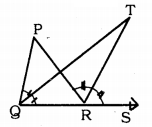Data: Arm QR of ∆PQR is produced upto S. Angular bisectors of ∠PQR and ∠PRS meet at T.
To Prove: ∠QTR = 12 ∠QPR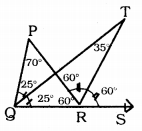Proof: Let ∠PQR = 50°, and ∠PRS = 120°
∠PQT = ∠TQR = 25°
∠PRT = ∠TRS = 60°
QR arm of ∆ PQR is produced upto S.
∴Exterior angle ∠PRS = ∠PQR + ∠QPR
120 = 50 + ∠QPR
∴ ∠QPR = 120 – 50
∠QPR = 70°
∴ ∠PRQ = 60°
Now, in ∆TRQ,
∠TQR + ∠TRQ + ∠QTR = 180°
25 + 120 + ∠QTR = 180°
145 + ∠QTR = 180°
∠QTR = 180 – 145
∴ ∠QTR = 35°
Now, ∠QTR = 35° ∠QPR = 70°
∠QTR = 702
∴ ∠QTR = 102 x ∠QPR.

All Chapter KSEEB Solutions For Class 9 Maths

—————————————————————————–

All Subject KSEEB Solutions For Class 9

*************************************************

I think you got complete solutions for this chapter. If You have any queries regarding this chapter, please comment on the below section our subject teacher will answer you. We tried our best to give complete solutions so you got good marks in your exam.

If these solutions have helped you, you can also share kseebsolutionsfor.com to your friends.

Best of Luck!!